# 会Python的淘宝商家可以横扫一切竞争对手，这就是会技术的魅力！（上）

## 前言

我虽然没做过电商，但是我买过他们的东西啊，所以就如淘宝上买的卖家，不管什么商品，卖家都是非常多，今天就教大家电商数据分析，如何找准自己商品的增长点才是最重要的！

# 先看下整体的一个步骤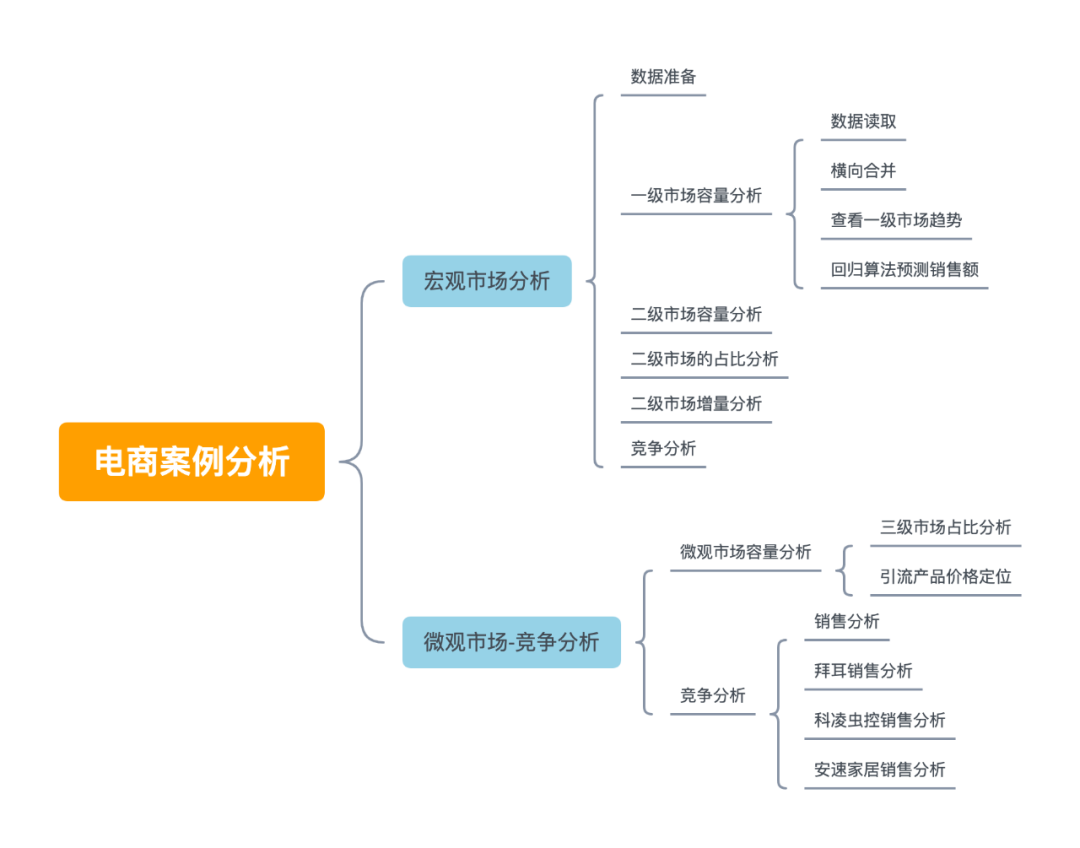## 电商案例分析

### 总体思路

1、了解行业与公司现状

• 行业现状与趋势，如涉及哪些业务

• 公司在市场中地位

2、沟通明确需求

• 头脑风暴，挖掘需求或瓶颈

• 多次沟通，最有价值的分析

3、最后用数据进行验证

### 导入相关模块

``````import numpy as np
import pandas as pd
import matplotlib.pyplot as plt
import seaborn as sns
import datetime
sns.set()
pd.set_option('float_format', lambda x: '%.2f' % x)

#显示中文
plt.rcParams['font.family'] = ['Arial Unicode MS']
plt.rcParams['axes.unicode_minus']=False
``````

## 宏观市场分析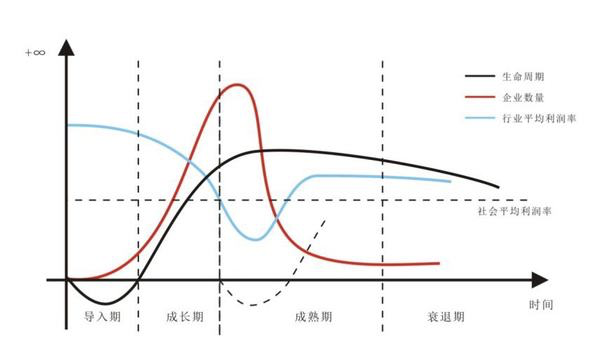1、起步期

2、成长期

3、成熟期

4、衰退期

### 数据准备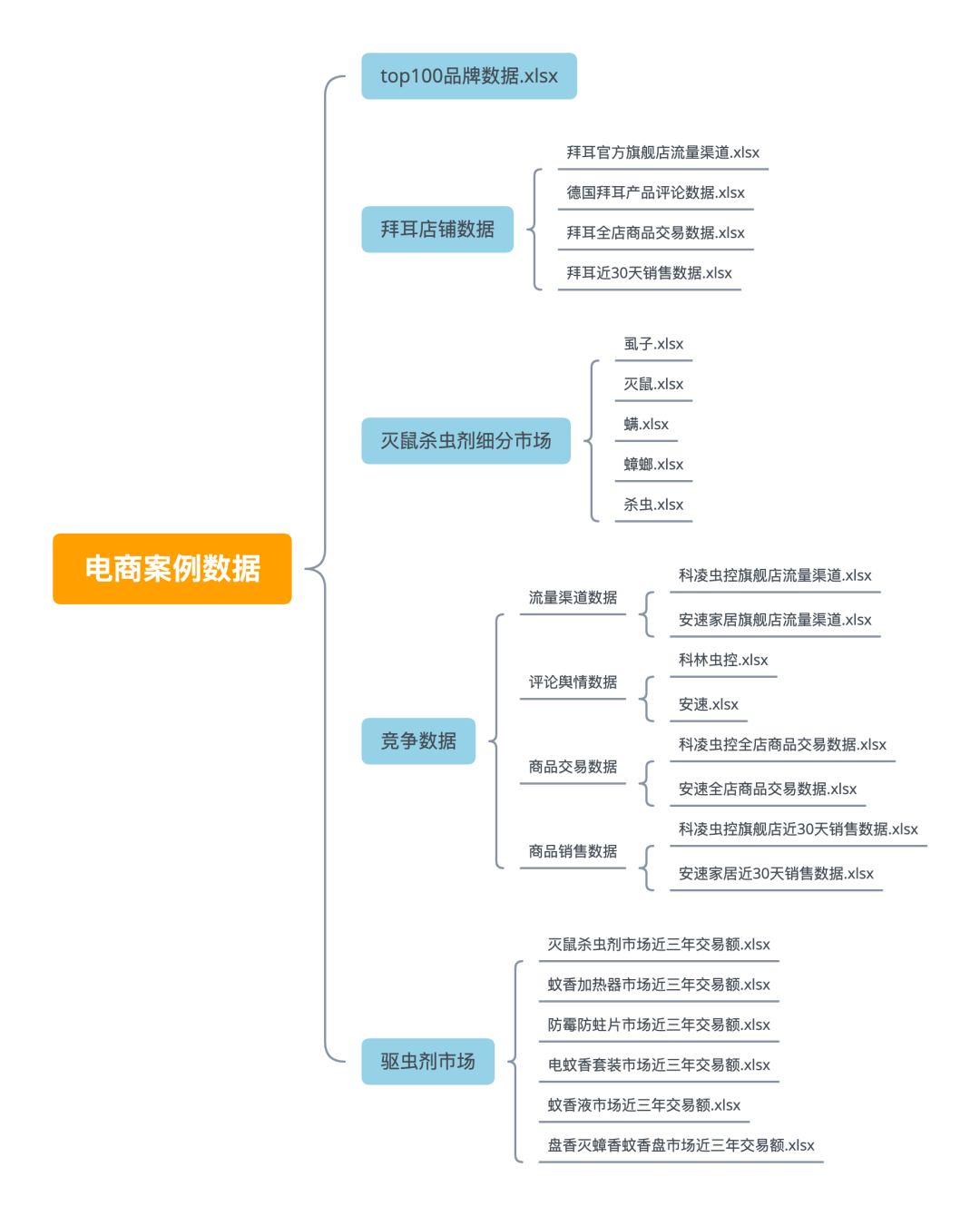### 一级市场容量分析

``````wxtz=pd.read_excel('./baier/电商案例数据/驱虫剂市场/电蚊香套装市场近三年交易额.xlsx')
``````

``````table = wxy
for i in [wxp,wxtz,ff,mssc,wx,jrp]:
table = pd.merge(table,i,how='inner',on='时间')
table.columns=['时间','电蚊香套装','防霉防蛀片','灭鼠杀虫剂','盘香灭蟑香蚊香盘','蚊香加热器','蚊香片','蚊香液']
``````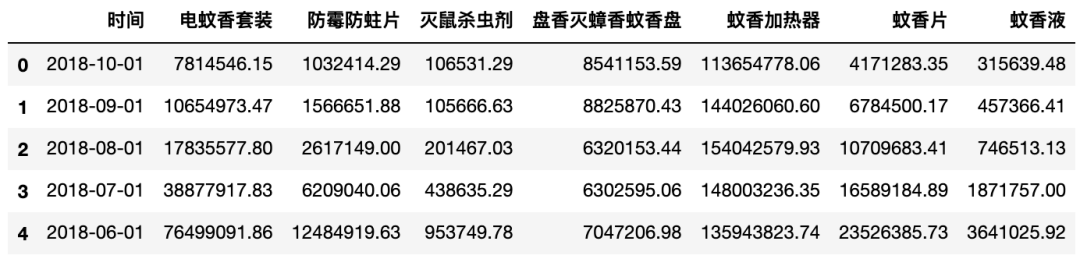``````table.set_index('时间',inplace=True)
table['一级市场销售总额']=table.sum(axis=1)
# table.一级市场销售总额.plot()
``````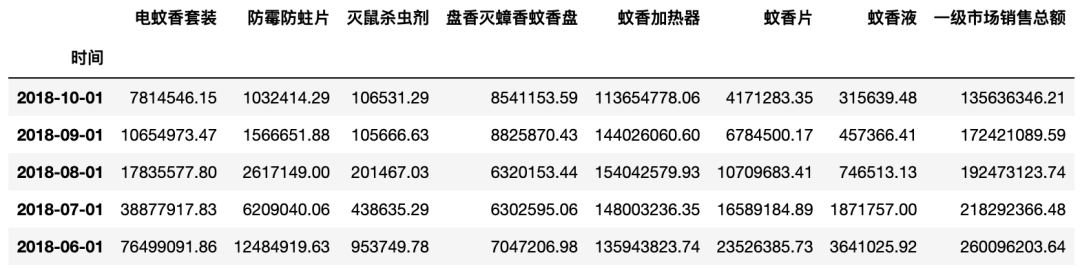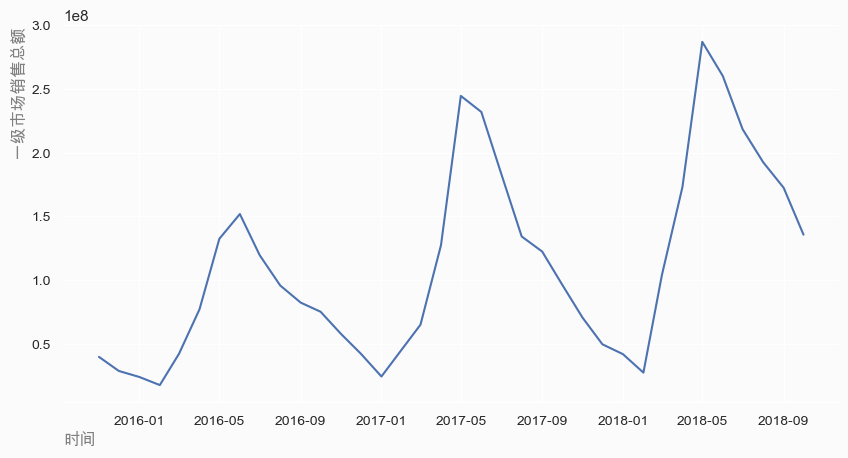• 一般作宏观市场容量分析，一般要求5年。

• 发现年度数据不规整，2018年11月、12月缺失，2015年只有11月、12月。

• 接下来用回归分别对18年11月、12月数据做出简单的预测。

``````table_02 = pd.concat([table['2015-11'],table['2016-11'],table['2017-11']],axis=0)
table_02
``````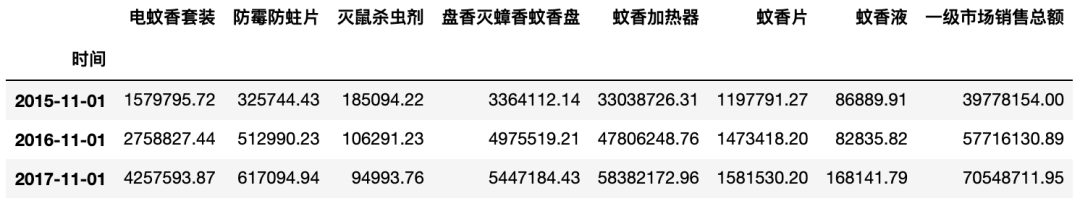``````from sklearn.linear_model import LinearRegression
y_11 = []
x_train=np.array([2015,2016,2017]).reshape(-1,1)

for i in table_02.columns:
y_train=table_02[i].values
clf = LinearRegression()
clf.fit(x_train,y_train)
y_pred = clf.predict(np.array([]).round(1))
y_11.append(round(y_pred,2))
y_11
``````
``````[5543203.83,
776627.04,
38692.61,
6678677.55,
71752495.99,
1801318.82,
193874.39,
86784890.23]
``````

``````date = datetime.datetime.strptime('2018-11-1','%Y-%m-%d')
y_11 = pd.Series(y_11,index=['电蚊香套装','防霉防蛀片','灭鼠杀虫剂','盘香灭蟑香蚊香盘',
'蚊香加热器','蚊香片','蚊香液','一级市场销售总额']
,name=date)
table_02.loc[date,:]=y_11
table_02
``````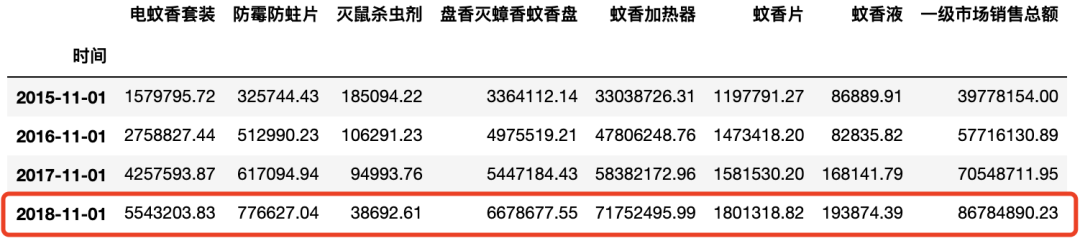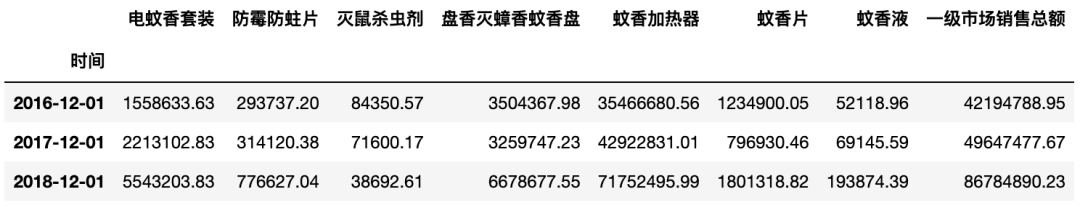### 二级市场容量分析

``````table_04 = pd.concat([table,table_02,table_03],axis=0)
# 根据索引排序
table_04 = table_04.drop_duplicates(
).sort_index(ascending=False).iloc[:-2,:]
``````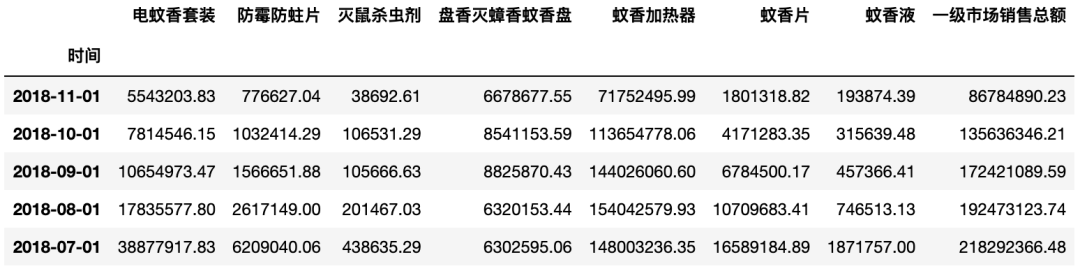``````# table_04.resample('Y')['一级市场销售总额'].sum().plot()
t4 = table_04.resample('Y')['一级市场销售总额'].sum()
``````
``````时间
2016-12-31    917966079.69
2017-12-31   1395872729.27
2018-12-31   1699011039.03
Freq: A-DEC, Name: 一级市场销售总额,
dtype: float64
``````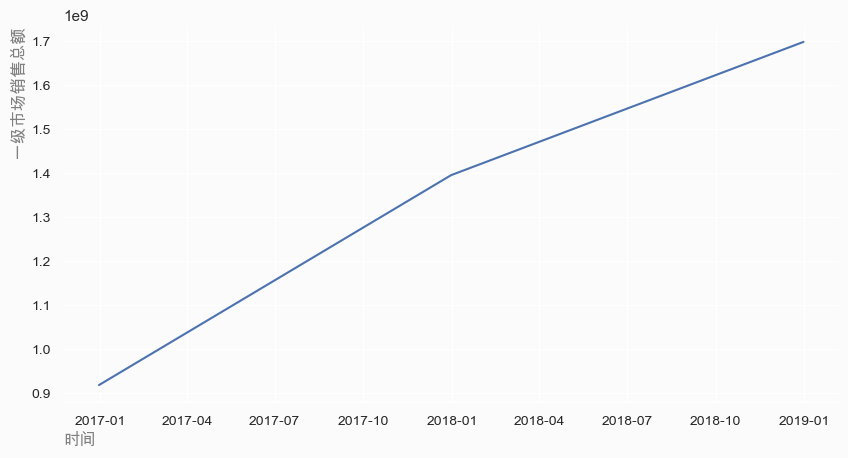``````table_05 = table_04.resample('Y').sum()
table_05
``````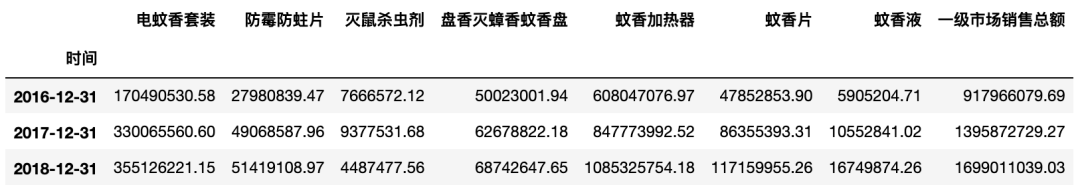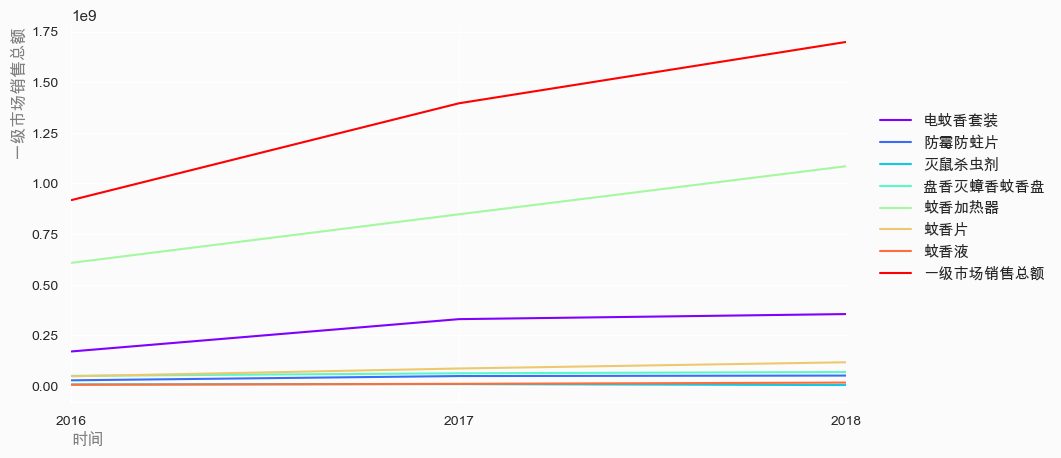• 二级市场里面，灭鼠杀虫剂市场销售额市场最大，增长幅度稳定

• 接下来对细分市场作分析，集中在灭鼠杀虫市场内，也是由于需求方产品线所处对二级市场。

• 灭蚊市场占比较大，增加产品多样性可以考虑灭蚊产品。

### 二级市场的占比分析

``````a=[]
for i in table_05.columns:
a.append(table_05[i]/table_05.一级市场销售总额)
table_06=pd.DataFrame(a,index=table_05.columns)
table_06.T
``````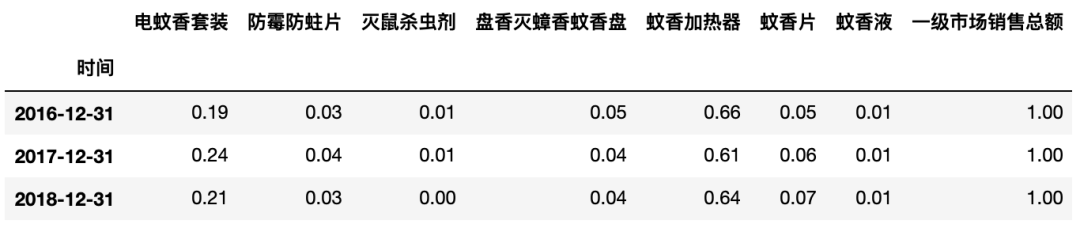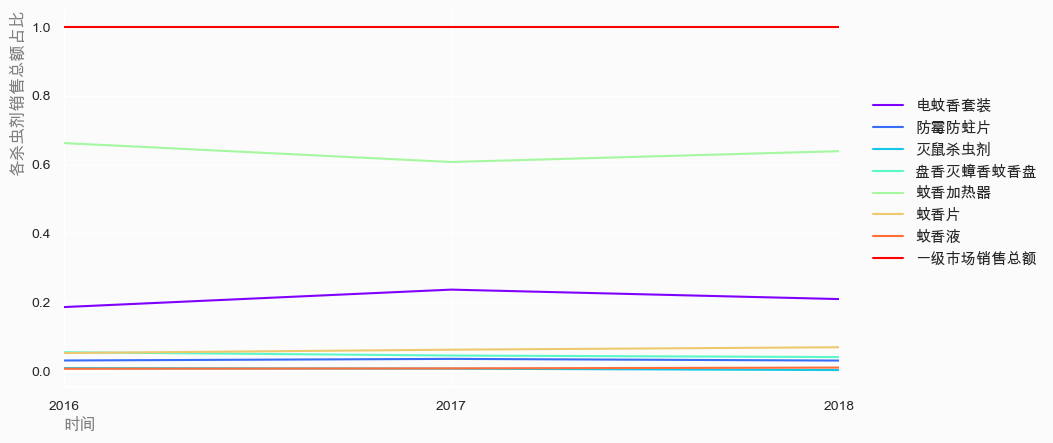• 灭鼠杀虫剂市场一直以来占领一级市场一级市场60%以上的市场份额。

• 其次是蚊香液产品，占据20%以上份额。

### 二级市场增量分析

``````incre_01=(table_05.iloc[1,:]-table_05.iloc[0,:])/table_05.iloc[0,:]
incre_02=(table_05.iloc[2,:]-table_05.iloc[1,:])/table_05.iloc[1,:]
incre=pd.DataFrame([incre_01,incre_02],index=['16-17年增幅',"17-18年增幅"])
incre
``````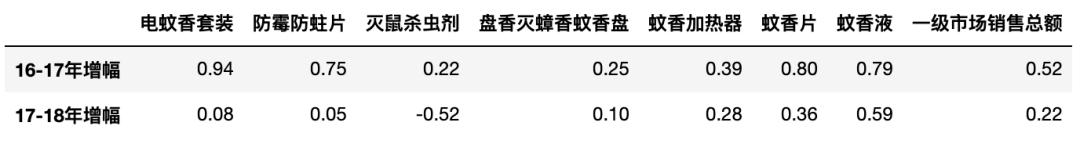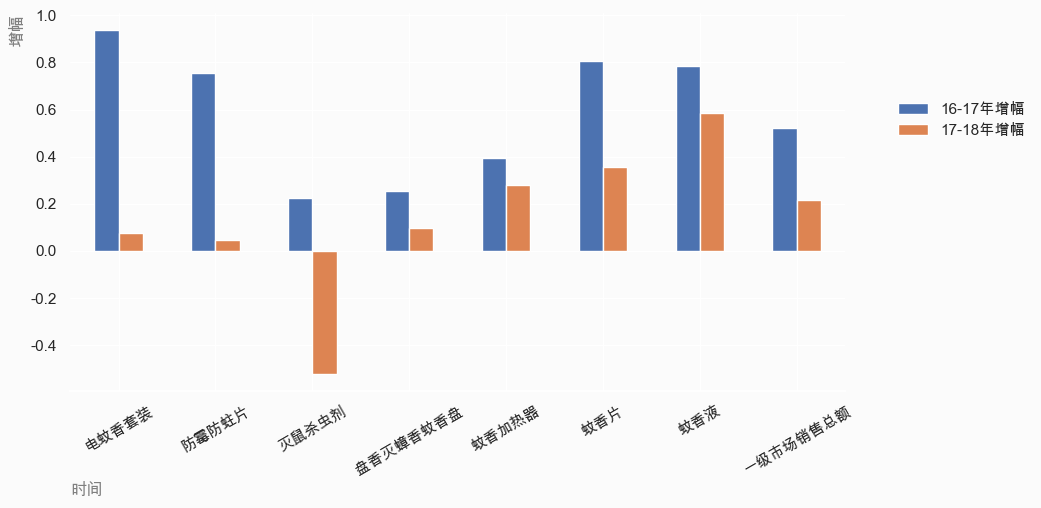• 一级市场容量的增幅确实是在放缓，放缓幅度较大。

• 二级市场容量有所放缓，幅度较小。

### 竞争分析

``````brand=pd.read_excel('./baier/电商案例数据/top100品牌数据.xlsx')
``````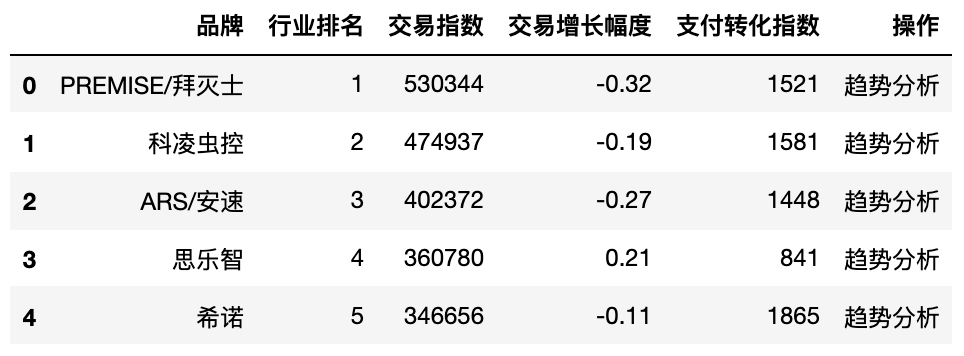``````brand=brand[['品牌','交易指数']]
brand.set_index('品牌',drop=True,inplace=True)
``````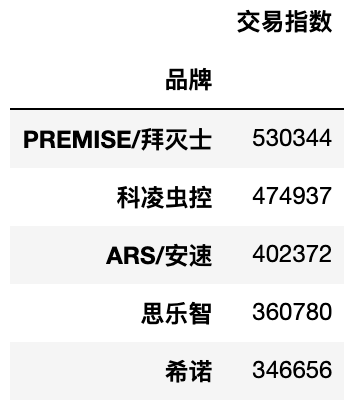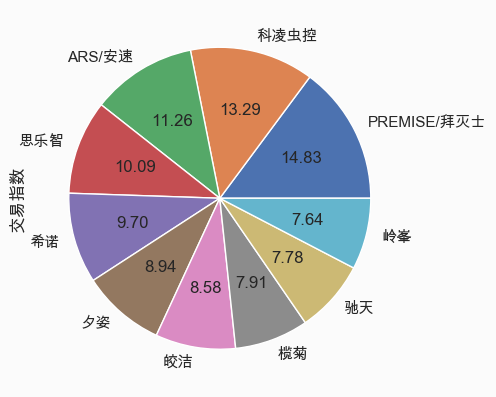## 微观市场-竞争分析

``````mm=pd.read_excel('./baier/电商案例数据/灭鼠杀虫剂细分市场/螨.xlsx')
``````
``````t_01=sz[["类别","预估销售额"]]
t_02=zl[["类别","预估销售额"]]
t_03=mm[["类别","预估销售额"]]
t_04=ms[["类别","预估销售额"]]
t_05=sc[["类别","预估销售额"]]
``````

### 微观市场容量分析

``````t = pd.concat([t_01,t_02,t_03,t_04,t_05])
t.set_index('类别',drop=True,inplace=True)
(t.groupby('类别').sum()).plot(kind='barh',
color=cmap,ax=ax)
``````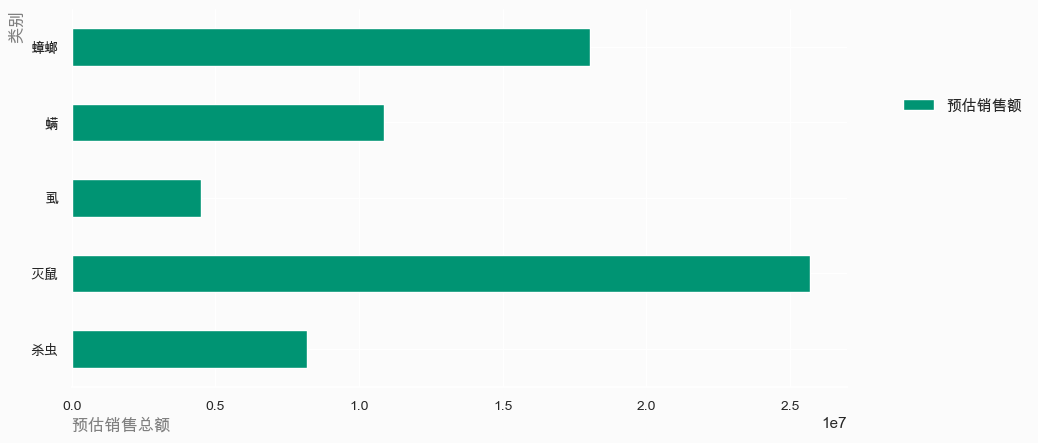• 在二级市场内，灭鼠产品的预估销售额是最大。

• 其次是才是灭蟑产品，目前拜耳的在驱虫剂市场的产品主要集中在灭蟑和杀虫。

• 可以考虑灭鼠和除螨的产品线。

``````t.groupby('类别').sum().sum(axis=0)
t = pd.concat([t_01,t_02,t_03,t_04,t_05])
(t.groupby('类别').sum()/t.groupby('类别').sum().sum(axis=0)
).plot(kind='barh',color=cmap,ax=ax)
``````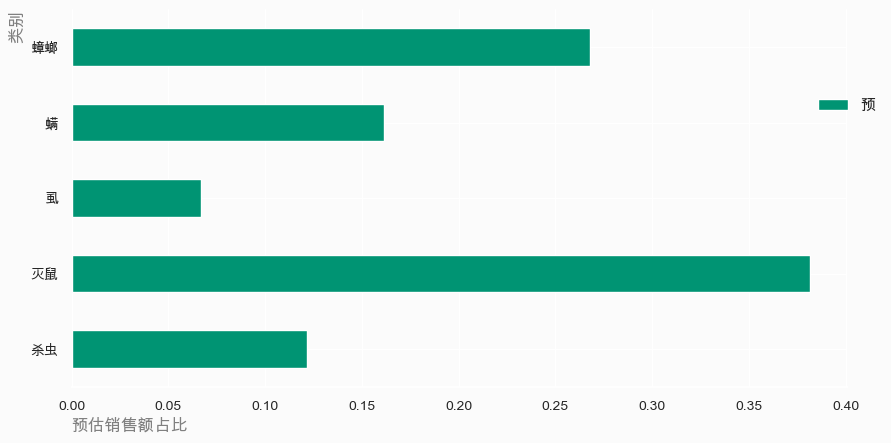• 拜耳主营业务市场，灭鼠和灭蟑产品占市场份额60%左右。

``````ms_02 = ms.loc[:,['类别','宝贝ID','售价','预估销售额']]
ms_02.describe()
``````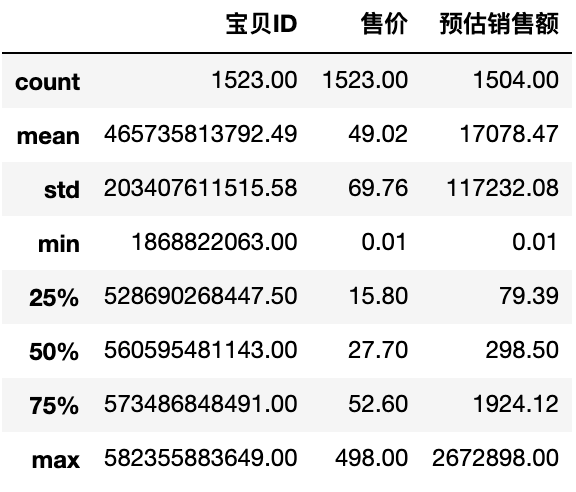``````bins_01 = [0,50,100,150,200,250,300]
labels = ['0-50','50-100','100-150','150-200','200-250','250-300']
ms_02['价格区间']=pd.cut(ms_02['售价'],bins=bins_01,labels=labels)
``````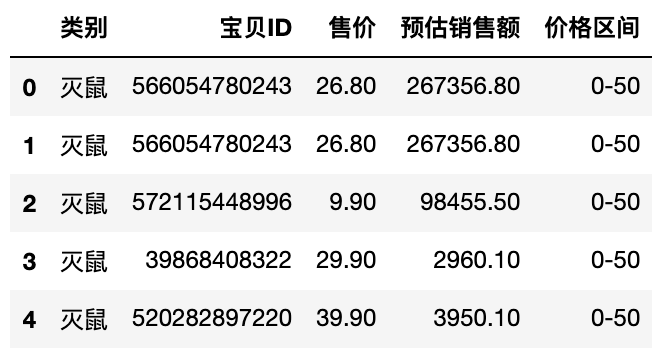``````ms_02.groupby('价格区间')['预估销售额'].sum().sort_values(ascending=False)
``````
``````价格区间
0-50      15162086.51
50-100     3335060.19
100-150    2758086.29
200-250    2743758.00
150-200     629813.00
250-300     237740.00
Name: 预估销售额, dtype: float64
``````

``````bins_02=[0,10,20,30,40,50]
labels_02=["0-10","10-20","20-30","30-40","40-50"]
ms_02['价格区间']=pd.cut(ms_02['售价'],bins=bins_02,labels=labels_02)
``````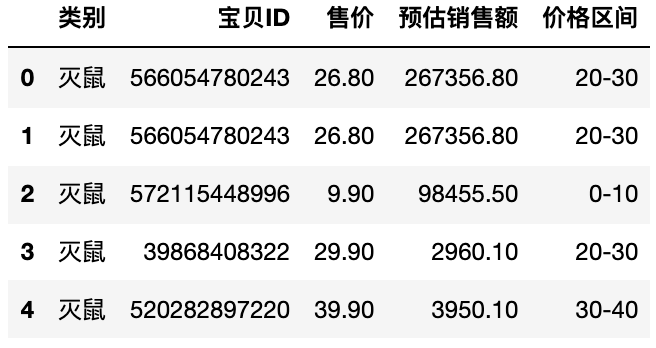``````ms_02.groupby('价格区间')['预估销售额'].sum().sort_values(ascending=False)
``````
``````价格区间
10-20   8102634.14
20-30   4969620.92
30-40   1240874.19
40-50    707568.49
0-10     141388.77
Name: 预估销售额, dtype: float64
``````

### 竞争分析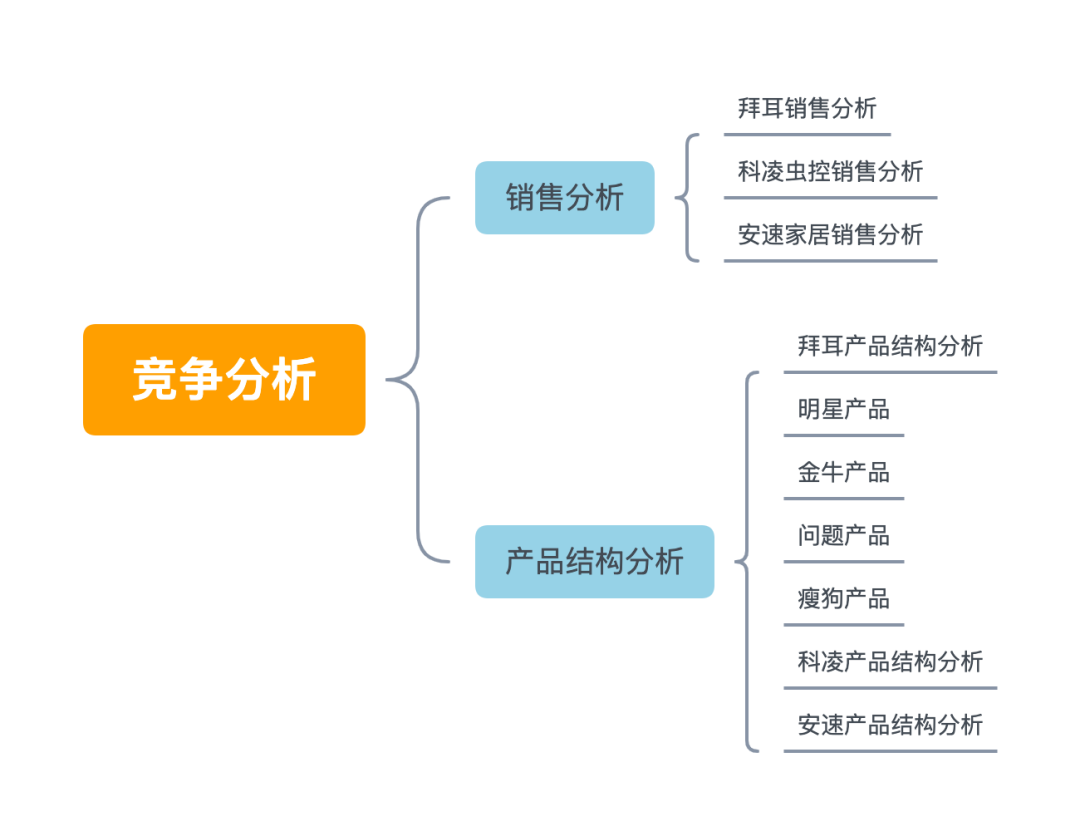``````安速家居 =pd.read_excel('./baier/电商案例数据/竞争数据/商品销售数据/安速家居近30天销售数据.xlsx')

``````

``````拜耳 = pd.read_excel('./baier/电商案例数据/拜耳店铺数据/拜耳近30天销售数据.xlsx')

``````
``````<class 'pandas.core.frame.DataFrame'>
RangeIndex: 15 entries, 0 to 14
Data columns (total 16 columns):
#   Column  Non-Null Count  Dtype
---  ------  --------------  -----
0   序号      15 non-null     int64
1   店铺名称    15 non-null     object
2   商品名称    15 non-null     object
3   商品ID    15 non-null     int64
4   主图链接    15 non-null     object
5   商品链接    15 non-null     object
6   商品原价    15 non-null     float64
7   商品售价    15 non-null     float64
8   30天销售量  15 non-null     int64
9   总销量     15 non-null     int64
10  类目      15 non-null     object
11  物理形态    11 non-null     object
12  型号      15 non-null     object
13  净含量     13 non-null     object
14  使用对象    15 non-null     object
15  销售额     15 non-null     float64
dtypes: float64(3), int64(4), object(9)
memory usage: 2.0+ KB
``````

``````baier = 拜耳[["商品ID",'使用对象',"商品售价","30天销售量","销售额"]]
``````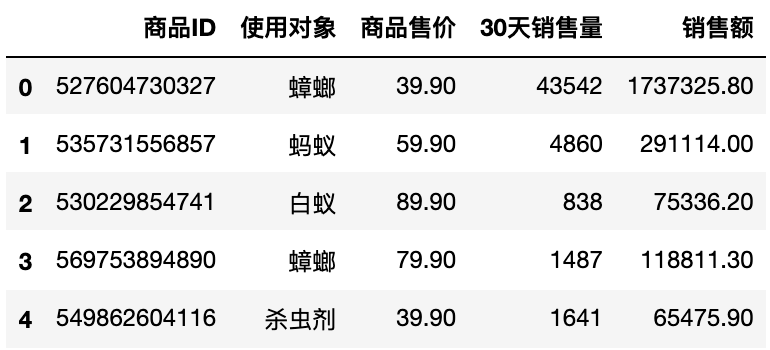``````cmap = [low_c,high_c]
(baier.groupby('商品ID')['销售额'].sum().sort_values(ascending=False)
).plot(kind='barh',color=cmap,ax=ax)
``````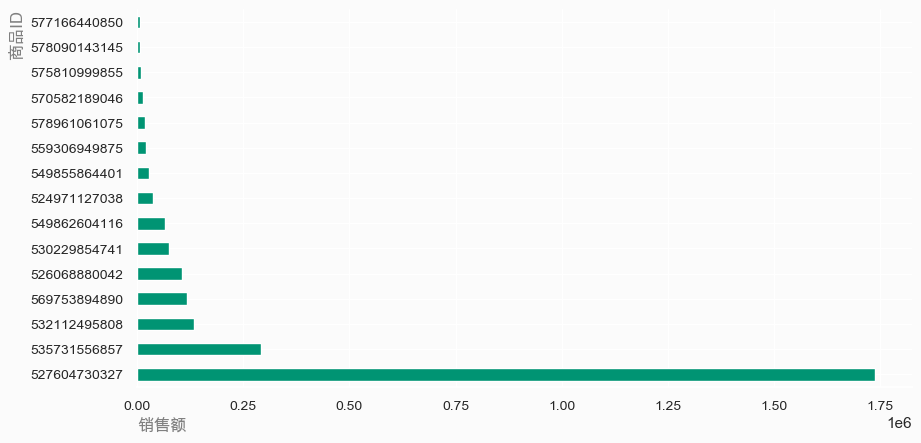``````baier.groupby('使用对象').sum().sort_values('销售额',ascending=False)
``````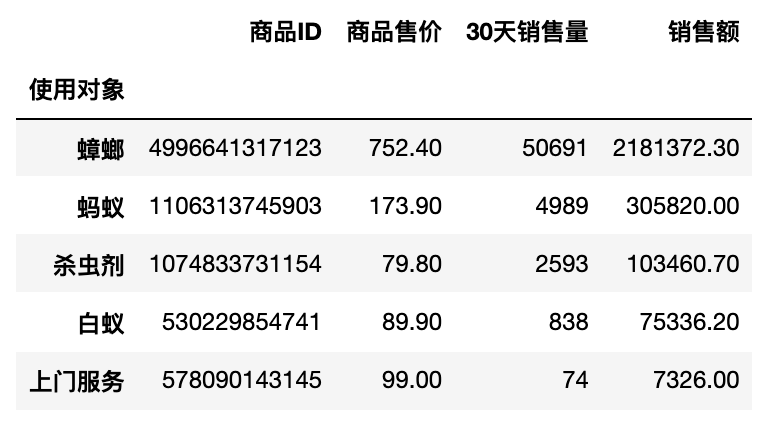• 灭蟑产品占拜耳的销售额最大的比重

• 其次是灭蚁等

``````keling = 科凌虫控[["商品ID",'适用对象',"商品售价","30天销售量","30天销售额"]]
keling.groupby('商品ID').sum().sort_values('30天销售额',ascending=False)
``````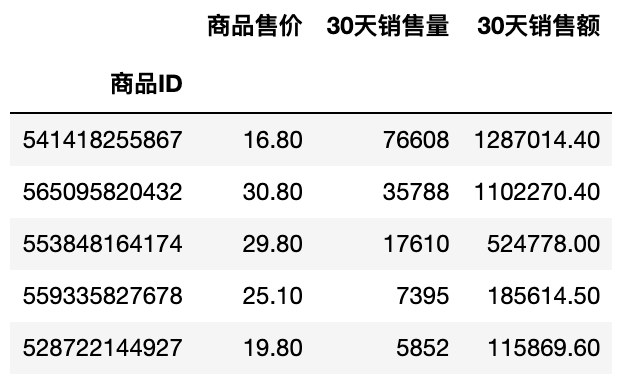``````keling.groupby('适用对象')['30天销售量','30天销售额'].sum().sort_values('30天销售额',ascending=False)
``````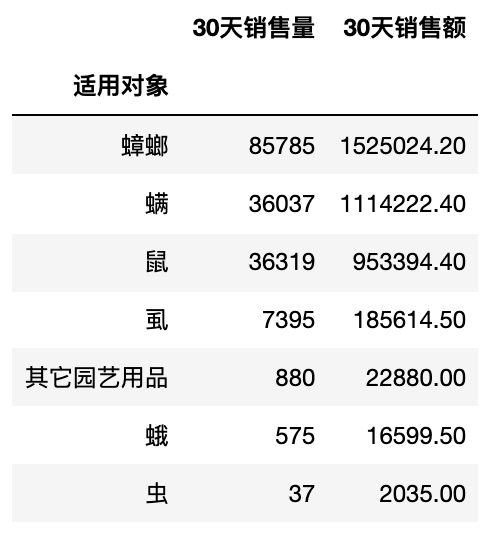• 热门产品（灭蟑）销售额与拜耳的差距不大

• 科凌的产品类目覆盖了二级市场里面主要的类目

``````ansu = 安速家居[["商品ID",'适用对象',"商品售价","30天销售量","30天销售额"]]
``````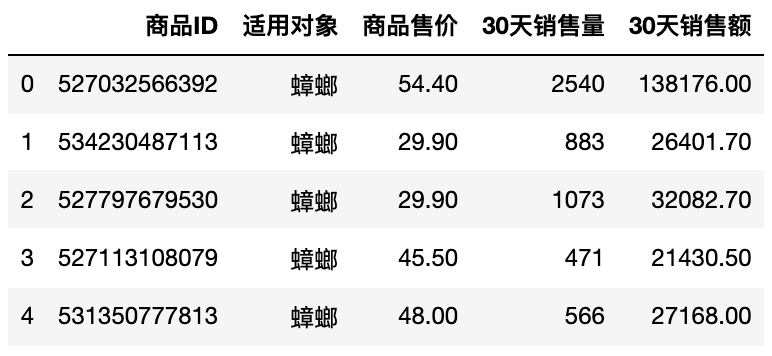``````ansu.groupby('商品ID')['30天销售量','30天销售额'].sum().sort_values('30天销售额',ascending=False)
``````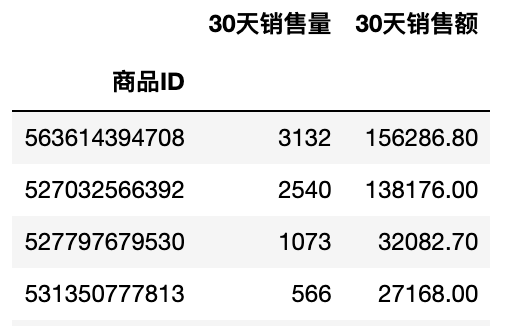``````ansu.groupby('适用对象')['30天销售量','30天销售额'].sum().sort_values('30天销售额',ascending=False)
``````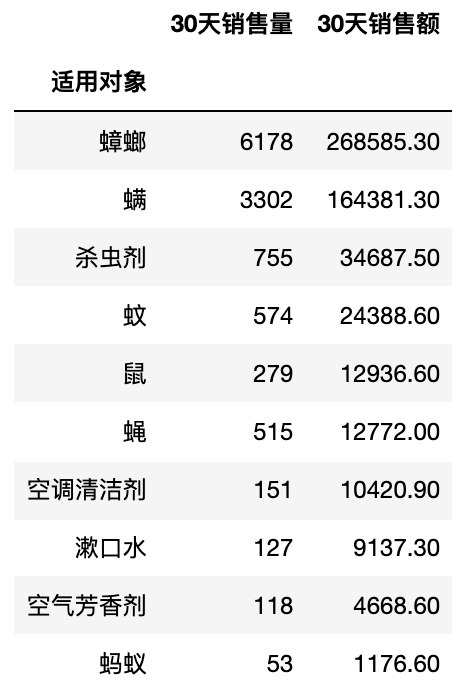• 热销产品的销售额不及拜耳与科凌

• 安速的产品类目广，基本覆盖了拜耳和科凌主营产品类目

• 拜耳热销产品主要集中在灭蟑，主要竞争对手（科凌、安速）在主营业务上构成竞争

• 主要竞争对手的产品多样化比拜耳做的更好

• 拜耳灭蟑产品只要竞争对手科凌，杀虫灭蚁产品主要挑战来源于安速

## 写在最后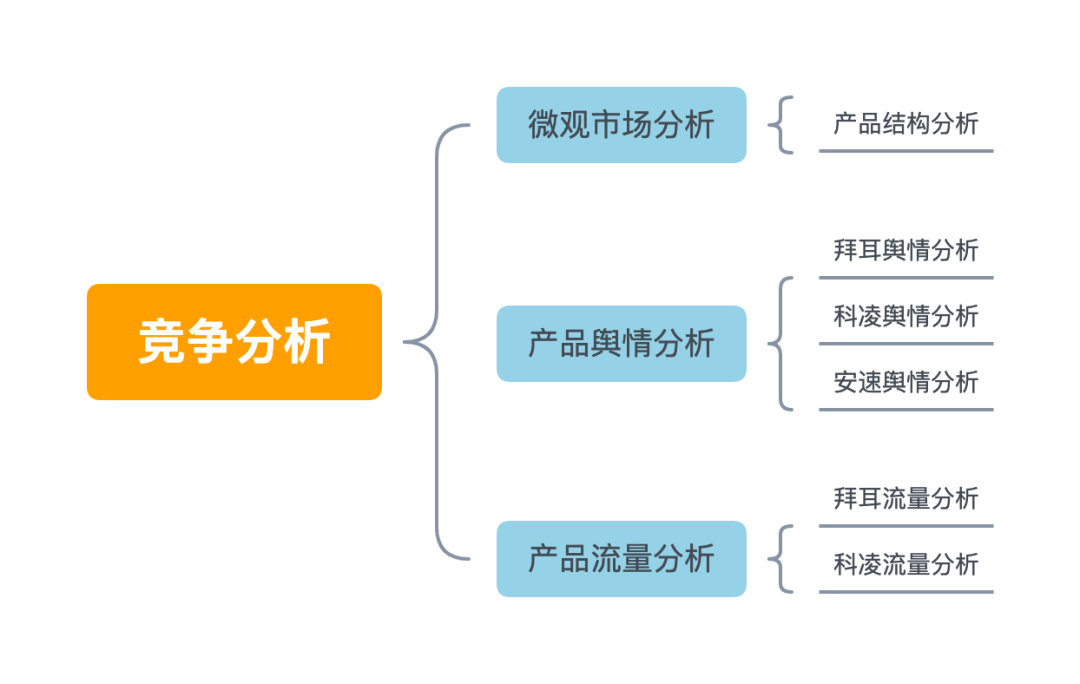### 热门文章## exe4j详细使用教程（附下载安装链接）## Android基础之WebView | 网络请求方式 | HttpURLConnection | OkHttp | 带实例

WebView 简介 一般用于加载一些网络界面Android内置webkit内核的高性能浏览器,而WebView则是在这个基础上进行封装后的一个 控件,WebView直译网页视图,我们可以简单的看作一个可以嵌套到界面上的一个浏览器控件&#xff01; 方法 WebChromeClient辅助WebView处理Javascript的…## AUTOSAR从入门到精通100讲（126）-浅谈车载充电系统通信方案

01 引言 本文深入研究车载充电系统策略，设计出一套基于电动汽车电池管理系统与车载充电机的CAN通信协议，可供电动汽车设计人员参考借鉴。 02 电动汽车充电系统通讯网络 电动汽车整车控制系统中采用的是CAN总线通信方式，由一个整车内部高速CAN网络、内部低速CAN网络和一个充电…## Kafka学习笔记十：kafka监控平台kafka-eagle的搭建和使用

1.下载压缩包 Download - EFAK 2.解压 # tar -zxvf kafka-eagle-bin-2.0.9.tar.gz 3.修改环境变量 #路径为解压路径 export KE_HOME/usr/local/software/kafka-eagle-bin-2.0.9/efak-web-2.0.9 export PATH\$PATH:\$KE_HOME/bin让配置生效 # source /etc/profile 4.修改kafka-e…## CMake（九）：生成器表达式## 【无标题】thinkphp6 文件上传## 47.第十章 网络协议和管理配置 -- 网络配置(八)

4.3.3 route 命令 路由表管理命令 路由表主要构成: Destination: 目标网络ID,表示可以到达的目标网络ID,0.0.0.0/0 表示所有未知网络,又称为默认路由,优先级最低Genmask:目标网络对应的netmaskIface: 到达对应网络,应该从当前主机哪个网卡发送出来Gateway: 到达非直连的网络,…## 元宇宙技术基础## 十进制正整数与二进制字符串的转换（C++）

Function one&#xff1a; //十进制数字转成二进制字符串 string Binary(int x) {string s "";while(x){if(x % 2 0) s 0 s;else s 1 s;x / 2;}return s; } Function two&#xff1a; //二进制字符串变为十进制数字 int Decimal(string s) {int num 0, …## [含lw+源码等]微信小程序校园辩论管理平台+后台管理系统[包运行成功]Java毕业设计计算机毕设## 【附章2Java面向对象编程】盘点关键字详解 this、super、final

❤写在前面 ❤博客主页&#xff1a;努力的小鳴人 ❤系列专栏&#xff1a;Java基础学习&#x1f60b; ❤欢迎小伙伴们&#xff0c;点赞&#x1f44d;关注&#x1f50e;收藏&#x1f354;一起学习&#xff01; ❤如有错误的地方&#xff0c;还请小伙伴们指正&#xff01;&#x1…## 树莓派驱动DHT11温湿度传感器

1&#xff0c;直接使用python库 代码如下 import RPi.GPIO as GPIO import dht11 import time import datetimeGPIO.setwarnings(True) GPIO.setmode(GPIO.BCM)instance dht11.DHT11(pin14)try:while True:result instance.read()if result.is_valid():print(ok)print(&quo…## ELK简介

ELK简介 ELK是三个开源软件的缩写&#xff0c;Elasticsearch、Logstash、Kibana。它们都是开源软件。不过现在还新增了一个 Beats&#xff0c;它是一个轻量级的日志收集处理工具(Agent)&#xff0c;Beats 占用资源少&#xff0c;适合于在各个服务器上搜集日志后传输给 Logstas…## Linux 基础## Windows2022 无线网卡装不上驱动## 【嵌入式面试宝典】版本控制工具Git常用命令总结## 用Postman生成测试报告